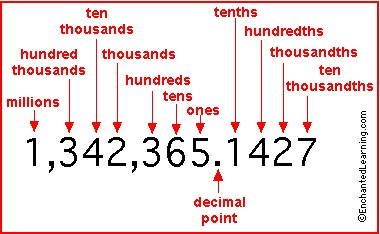# How Do You Write In Standard Form?

## 1 Answers

Oddman answered
It depends on what you are writing.

A linear equation -- ax + by = c (the leading non-zero coefficient is positive)
A quadratic equation -- ax^2 + bx + c = 0
A number (where "standard form" is scientific notation) -- a.b*10^c where a > 0, b may be a string of digits, a or c or both may be negative.
The equation for a circle -- (x-h)^2 + (y-k)^2 = r^2
A number, where "standard form" is not scientific notation ...thanked the writer.

## Answer Question